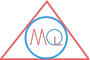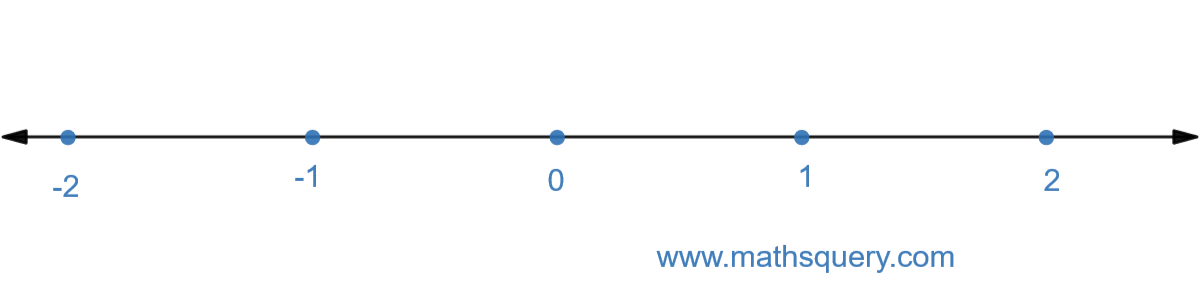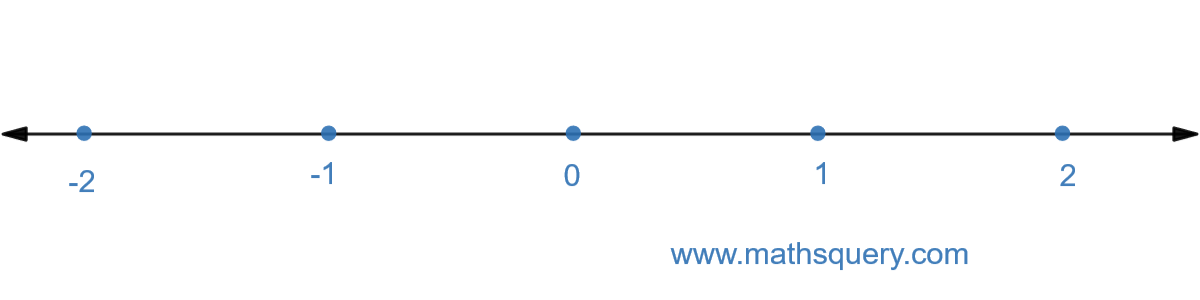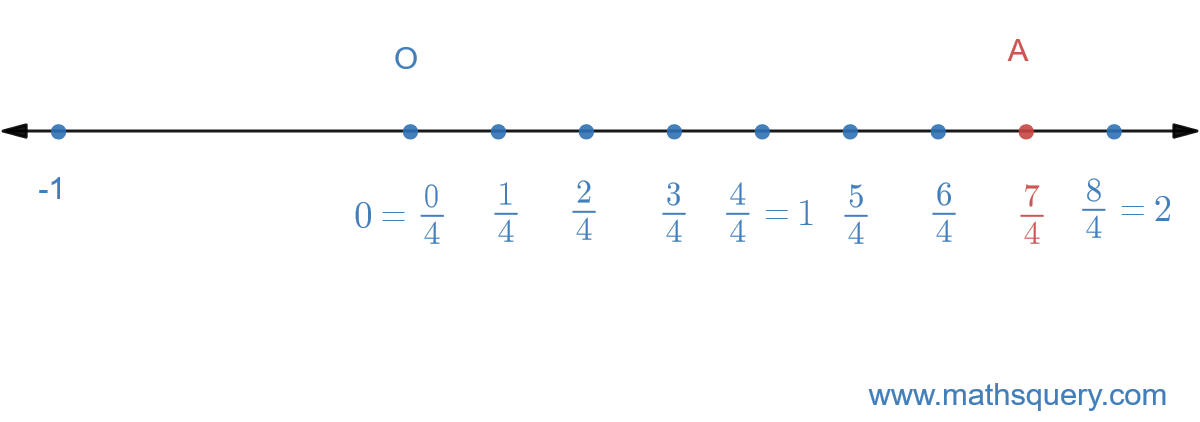# Rational Number and its Representation on Number Line

## Introduction

To start with what are rational numbers and how do we write them, there is a brief introduction about them here.
We will discuss more about them here and learn if we can represent them on the number line and how.

## What is rational number?

A number which can be written in the form of $$\frac{p}{q}$$, where p and q are integers and $$q \; \neq \; 0$$.

Example

$$\frac{1}{2}$$, $$\frac{6}{7}$$, $$\frac{9}{10}$$, $$\frac{3}{10}$$ etc.

Note

Even 0 is also a rational number as 0 can be written as $$0 \; =\;\frac{0}{1}$$

## How to represent rational number on number line.

Rational number can be written on number line same as usual numbers.
The following steps will help in representing a rational number on number line.

Step 1
Draw a number line with positive numbers on the right hand side and negative number on the left hand side of 0.
Step 2
Divide distance between 0 and 1 into n equal points.
Mark the points as $$\frac{1}{n}$$, $$\frac{2}{n}$$, $$\frac{3}{n}$$, $$\frac{4}{n}$$ etc.

Let’s learn the above steps with an example.

Example

Example 1: Represent $$\frac{3}{7}$$ on number line.
Step 1
Draw a number line with positive numbers on the right hand side and negative number on the left hand side of 0.Step 2
Here, n = 7.
So, divide distance between 0 and 1 into 7 equal points.
Length of each part between two adjacent points is $$\frac{1}{7}$$.
Now, start from 0 and mark the point $$\frac{3}{7}$$ on number line.So, OA = $$\frac{3}{7}$$

Example 2: Represent $$\frac{7}{4}$$ on number line when value of denominator is less than value of numerator.
Step 1
Draw a number line with positive numbers on the right hand side and negative number on the left hand side of 0.Step 2
Here, n = 4.
So, divide distance between 0 and 1 into 4 equal points and do the same from 1 to 2, i.e. divide them into 4 equal parts.
Length of each part between two adjacent points is $$\frac{1}{4}$$.
Mark the point $$\frac{7}{4}$$ on number line.So, OA represents $$\frac{7}{4}$$